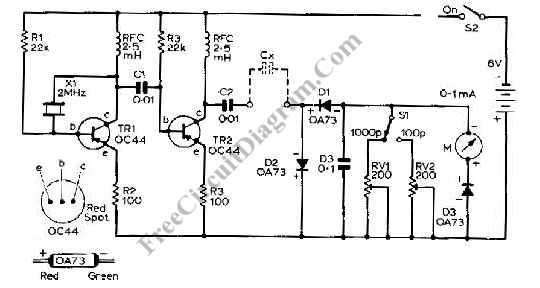# Capacitance Meter Circuit Using TransistorsThis capacitance meter circuit is similar with previous meter circuit, but it uses transistors rather than logic gates. Here is the schematic diagram:You can see the circuit uses pnp transistor, you can use almost any pnp transistors with frequency bandwidth og 100MHz and collecttor current handling capability of 100 mA to replace the transistor if you can find the exact type as shown in the schematic diagram.